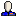Josiah (akachouyu_31) has posted an update to our paper, now called The Weighted Maximum-Mean Subtree and Other Bicriterion Subtree Problems. As the new title implies, we can now solve a much broader class of optimal subtree problems, it connects more directly to the parametric optimization thread that's been running through quite a few of my papers, and I think also that the writing is much improved.

I think it's an interesting problem both theoretically and for its applications, which can be easily motivated in terms of optimizing return on investment in tree-structured decision processes, but you'll have to read the paper for the details. Instead, I wanted to briefly discuss an odd three-way complexity variation involving negative numbers in the title problem. It's sort of like what happens with shortest paths, where Dijkstra works for positive arc lengths, Bellman-Ford works but is slower for negative arc lengths but no negative cycle, and shortest simple paths are NP-complete when there may be negative cycles.

So, suppose you have a rooted tree, in which the nodes have both an investment cost and an eventual profit, both in dollars; you want to choose a subtree containing the root that maximizes the percentage return on investment, or equivalently the weighted average of the chosen nodes' profit/cost ratios, where each node's ratio is weighted by its cost. The result in the first version of the paper was that the subtree maximizing this weighted average can be found in linear time if the costs are all positive. If some subtrees may have negative total cost, the problem is NP-complete via a straightforward reduction from subset sum, as we show in the new version of the paper. But the main new result of the update is an algorithm that, when applied to this weighted average problem, allows individual nodes to have negative costs (that is, they pay you to invest in them rather than vice versa) as long as the total weight of any subtree containing the root is positive. As with Bellman-Ford, this limited negativity slows us down but doesn't stop us solving the problem: we have an O(n log n) algorithm.

Is the extra logarithmic factor necessary for the limited-negativity version of the problem? I don't know, but it is necessary for the parametric linear optimal subtree problem we use as the basis for our algorithm, since we have a reduction from sorting to it...